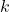# A class of estimators of regression coefficient for sign change problem in measurement errors

Srivastava and Shalabh (1997a, Journal of Econometrics) proposed a class of
Stein-like consistent estimators for estimating the slope coefficient in a single explanatory
variable ultrastructural model. This paper studies the sign reversal
problem of this class of estimators and proposes an alternative class of improved
estimators along the lines of the double-class estimators of Ullah and Ullah
(1978,\textit{ Econometrica}) that overcomes this problem. Large sample asymptotic
properties of the proposed estimators are studied for the case where the distributions
of the measurement errors are not necessarily normal.

Fulltext